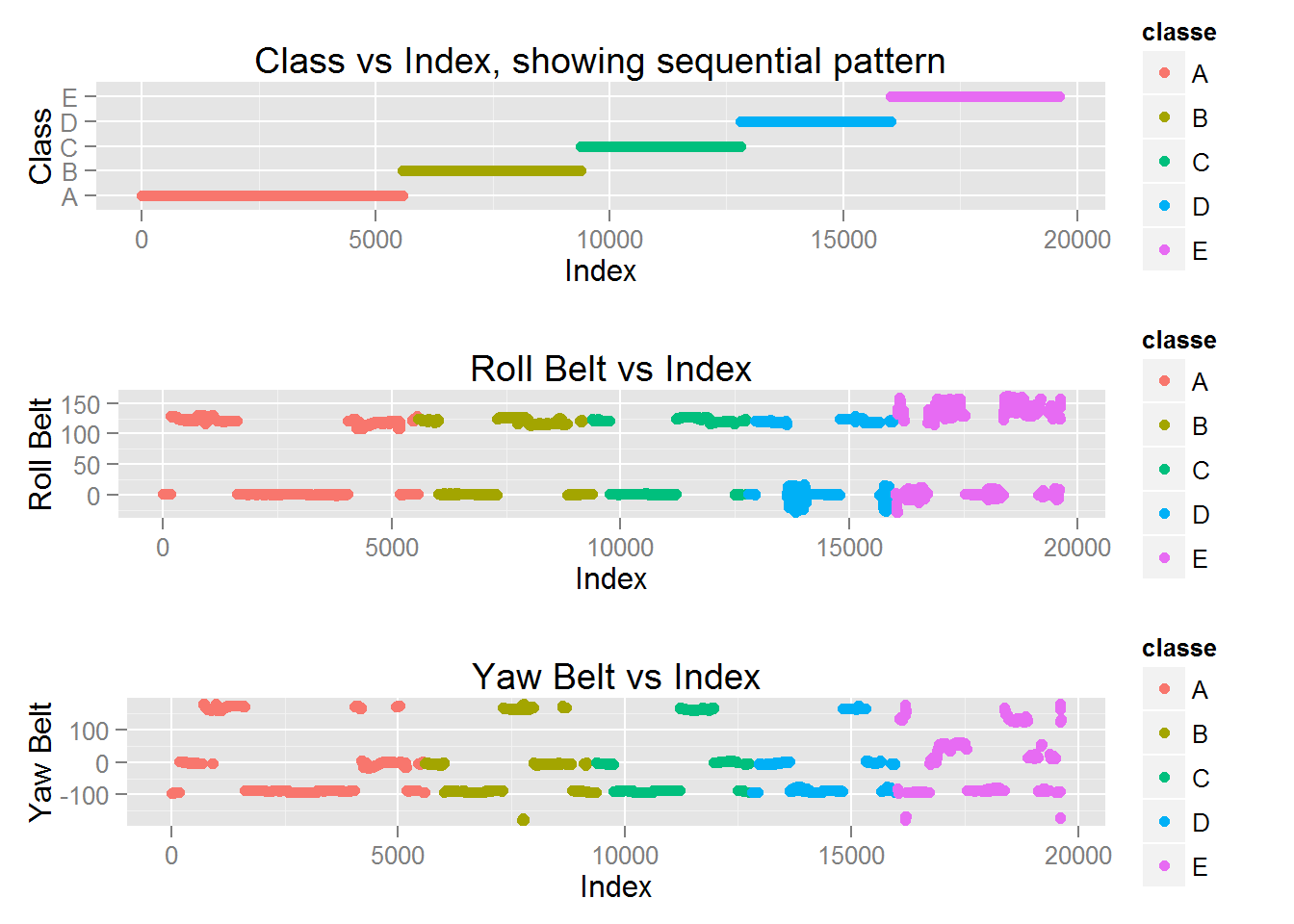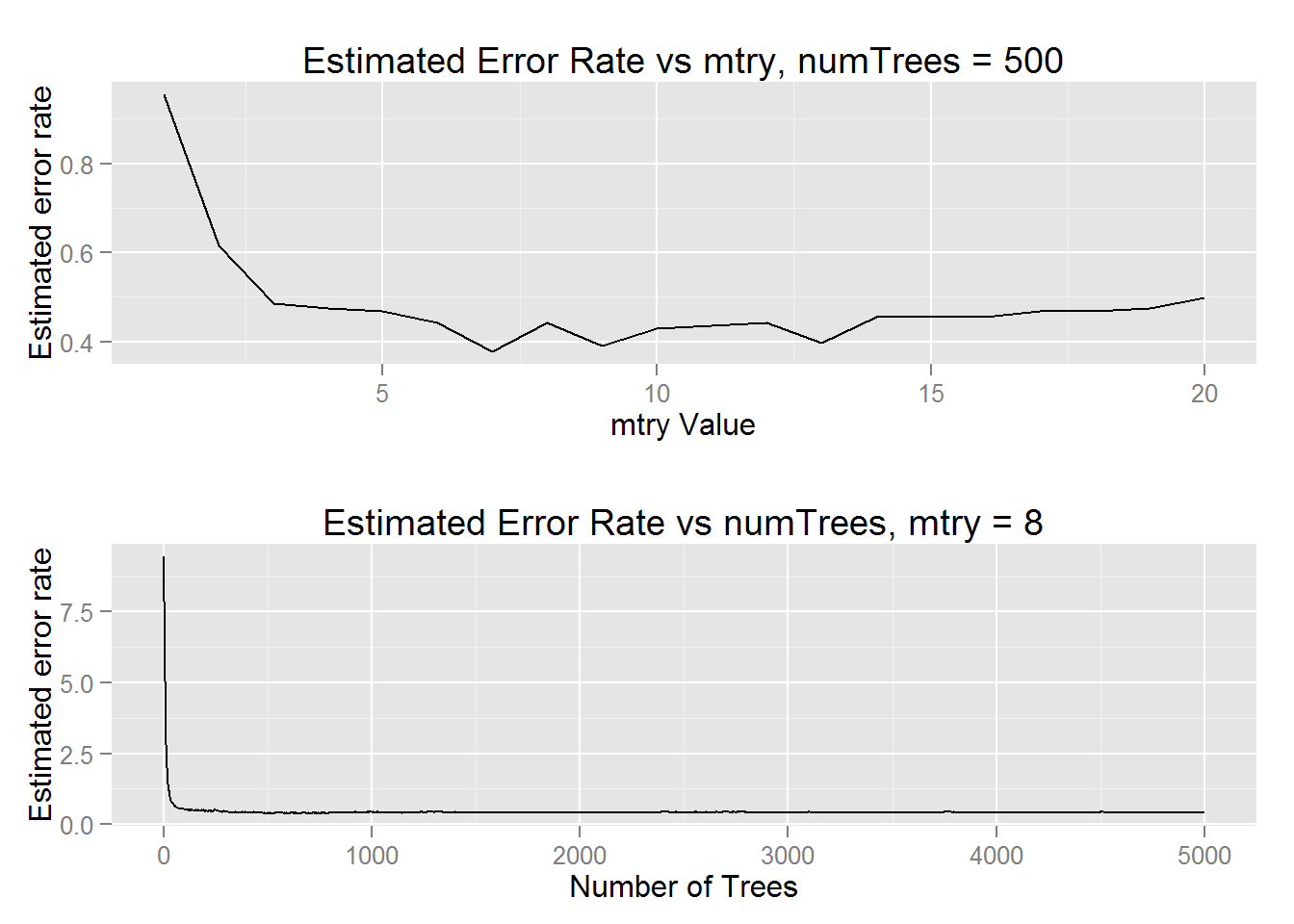# Predicting Exercise Form via accelerometer data

### Synopsis

We analyze a data set containing accelerometer measurements by which a type of dumbbell curl is classified. With over 19000 observations in our training set, 159 predictors each, and 5 potential classifications, model choice is a large factor in predictive performance. Using a random forest implementation, we achieve over 99% accuracy in our results after validating with a 4000 sample set.

``````training <- read.csv("pml-training.csv", na.strings = c("NA", ""))
testing <- read.csv("pml-testing.csv", na.strings = c("NA", ""))``````

Our data has many variables with 90%+ NA’s or blank entries. We remove them using the code below, and create a validation set sampling 4000 instances from our training set without replacement.

``````training <- training[, colSums(is.na(training)) == 0]
testing <- testing[, colSums(is.na(testing)) == 0]
validation <- sample_n(training, size = 4000)
training <- training[-validation\$X,]``````

### Exploratory Data Analysis

We use the random forest classification model, which will draw n = 500 bootstrap samples from the training data before growing a classification tree and choosing the best option among m = 8 predictors at each node. The m = 8 is standard for classification, given that we have 60 predictors after removing NA’s, round(sqrt(60)) = 8. It will then aggregate all results to deliver the majority vote classification value among all the trees.

We naively include all possible predictors in our first iteration and then examine the importance of particular variables.

``````fit.rf <- randomForest(classe ~ ., data = training, ntree = 500, mtry = 8, importance = TRUE)
fit.rf``````
``````##
## Call:
##  randomForest(formula = classe ~ ., data = training, ntree = 500,      mtry = 8, importance = TRUE)
##                Type of random forest: classification
##                      Number of trees: 500
## No. of variables tried at each split: 8
##
##         OOB estimate of  error rate: 0.01%
## Confusion matrix:
##      A    B    C    D    E class.error
## A 4420    0    0    0    0   0.0000000
## B    1 3007    0    0    0   0.0003324
## C    0    0 2703    0    0   0.0000000
## D    0    0    0 2612    0   0.0000000
## E    0    0    0    0 2879   0.0000000``````
``head(sort(fit.rf\$importance[,6], dec = TRUE))``
``````##                    X       cvtd_timestamp raw_timestamp_part_1
##              0.45459              0.21611              0.10218
##           num_window            roll_belt    magnet_dumbbell_y
##              0.05887              0.04933              0.03844``````

We find that the sequential X variable, as well as generic timestamps and other non-accelerometer data are having a large effect on our classification, and we would like to remove them to preserve generalizability in future accelerometer datasets. In addition, our classification is estimating a 0% error rate, hinting that there is likely overfitting going on due to the sequential nature of the indices and classification types.

We confirm this sequential bias in the following plot, and also examine some of the highest influencing predictors:There is a reasonable pattern to draw inference from in the roll and yaw belt data, especially with regards to the D and E class.

### Cleaning Data Redux

After evaluating some influential variables, we decide to minimize our features only by removing the qualitative predictors and leaving all accelerometer values.

``````training <- training[,8:length(training)]
testing <- testing[,8:length(testing)]
validation <- validation[,8:length(validation)]``````

### Predictive Modeling

With 52 predictors, we evaluate the best performing mtry value for our random forest model. As before, we expect round(sqrt(52)) = 7 to be close to the ideal value. We take the training set’s ideal value and use it to examine the effect of increasing the amount of trees (creating a larger ensemble).Using mtry = 8 and numTrees = 1000 seems to be near the ideal value for our given data set, so we perform our final data classification modeling using these values with the following code:

``fit.rf.final <- randomForest(classe ~ ., data = training, ntree= 1000, mtry = 8, importance = TRUE, keep.forest = TRUE)``

### Results

Given our final model’s out of bag error calculation, we estimate our out of sample error to be about 0.4%, or in other words, 99.6% accuracy .

``````valid.results <- predict(fit.rf.final, validation[-length(validation)])
orig <- dplyr::select(validation, classe)[,1]
accuracy_valid <- sum(valid.results == orig)/length(valid.results)*100``````

We use our random forest model to predict the values of our 4000 samples in our validation set and achieve an impressive 99.975% accuracy on our prediction, using only accelerometer data, with the most influential predictors listed below:

``````##         roll_belt          yaw_belt      roll_forearm magnet_dumbbell_y
##           0.13448           0.12344           0.12181           0.11988
## magnet_dumbbell_z magnet_dumbbell_x
##           0.11636           0.09554``````

We can see that roll_belt and yaw_belt remain some of the strongest predictors, as we found in the early stages of analysis.

In sum, the random forest machine learning algorithm performs quite well on this type of data, achieving about 99% accuracy in largely human-removed analysis, given qualitative, non-generalizable predictors and biases are removed.

In addition, the same prediction model was applied to the testing set and achieved 100% accuracy.